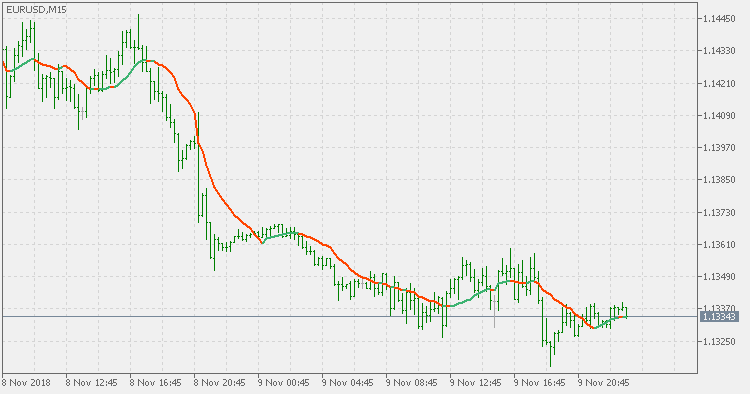Interesting script?
So post a link to it -
let others appraise it

You liked the script? Try it in the MetaTrader 5 terminal# Range weighted average - indicator for MetaTrader 5

Views:
1405
Rating:
Published:
2018.11.11 18:27

Theory :

Almost all averages can be made as an average with a generalized formula that could be simply written down as : sum of price*(something) / sum of (something). The usual averages that we have are simple moving average (SMA, where the (something) is always equal to 1), linear weighted moving average (LWMA, where the (something) depends on the calculation length), volume weighted average (where the (something) depends on the current volume) and some more ...

This version :

This version is using the range (high-low) of the bar it is calculated for as the weight. That way the bars with higher volatility are getting more emphasized and the response to price changes at those bars is accelerated. Basically, as almost all weighted averages, it is similar to simple moving average with an important difference at the times of high ranges where this indicator is much "faster" than the SMA

Usage :

You can use the color changes of this indicators as signalsFiltered WPR

Filtered WPR (Williams Percent Range)Filtered averages

Filtered averagesRange weighted average MACD

Range weighted average MACDRange weighted EMA

Range weighted exponential moving average (EMA)